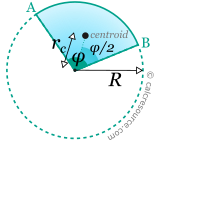## Properties of a Circular sector

This tool calculates the basic geometric properties of a circular sector. Enter the shape dimensions R and φ below. The calculated results will have the same units as your input. Please use consistent units for any input.

 R = φ = deg rad Geometric properties: Area = Perimeter = Arc length = rc =